# Team:British Columbia/modeling description

### Introduction

We developed a mathematical model that describes the dynamics of biofilm structure (in terms of bacterial population size) and the interactions among major components such as the engineered phage and Dispersin B (DspB) protein. We used numerical simulations to predict the impact of phage and DspB release on the biofilm. We also investigated the weight of each parameter with regards to the design of our system using sensitivity analyses. We implemented our model in a Java program called PhilmIt-v1. Our model can be used as a tool for designing genetically engineered phage-biofilm systems.

### Basic Biofilm Geometry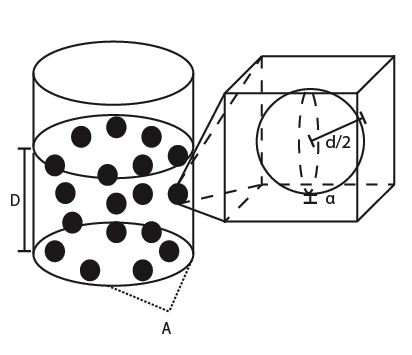Figure 1: Schematic diagram of the biofilm structure. A cylindrical (or rectangular) container of depth, D, and cross-sectional area, A, holds the biofilm mass. Each bacterium occupies a spherical volume of diameter, d, corrected by a constant representing the space filled by surrounding EPS, α (see zoom-in).

The biofilm system assumes a simple planar geometry characterized by depth, D, and cross-sectional area, A (Figure 1). The density and distribution of the biofilm bacterial population (B), extracellular polymeric substance (EPS), and dissolved components (e.g. AIP and metabolites) are uniform throughout the biofilm structure. Assuming that each biofilm bacterium occupies a spherical volume of diameter, d, and the surrounding EPS extends this volume by a constant, α, each bacterium takes up a cubic volume of (α + d)3, we can estimate the total biofilm population: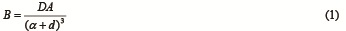The carrying capacity determines the maximum biofilm thickness, and therefore can be coarsely estimated by letting D equal to the maximum thickness in Equation 1.

### Biofilm Bacteria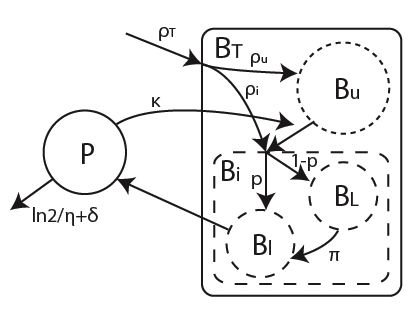Figure 2: Flow diagram indicating the interactions among the core components of the biofilm system. The total biofilm population, BT, is divided into subpopulations (inside the rounded box). The phage particle population, P, directly interacts with only the subpopulation infected with latent phage, BL.

The total bacterial population, BT, is divided into two subpopulations: 1) the carrier bacteria, Bi, which are infected with the engineered phage and 2) the non-carrier bacteria, Bu, which are uninfected but susceptible to phage infection upon exposure. The total population, BT, undergo logistic growth at rate ρT limited by the carrying capacity, K; the uninfected, Bu, and infected, Bi, subpopulations grow at rates ρu and ρi, respectively. We separate the carrier bacteria subpopulation further into two subpopulations: 1) the bacteria infected with the phage in latent phase, Bl, and 2) those infected with the phage in lytic phase, BL. The flow diagram in Figure 2 summarizes the hierarchical relationships among components of the biofilm population.

### Phage Particles

Initially, only the engineered S. aureus bacteria will be introduced to the biofilm. In response to the presence of AIP, they will generate and release the first batch of phage particles. A proportion of these phage particles, κ, will successfully infect and integrate its genetic information into the host genome. Once infected, a bacterium is subject to lysis probability of λ; upon lysis, the bacterium will release R number of phage particles. The dynamics of the phage particles is also governed by half-life, t1/2, and follows this differential equation, where δ is the portion of phage remaining in the biofilm structure after diffusion (described in detail in next section):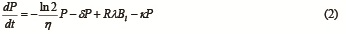### Phage Diffusion and Invasion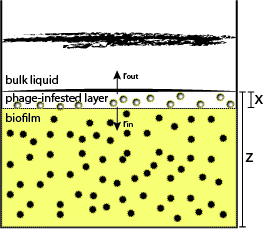Figure 3: Schematic diagram of the phage invasion process. Phage particles move into the biofilm structure via simple diffusion. Lysis of infected host cells in the phage-infested layer (indicated by a thickness of x) produces new pools of phage particles which undergo diffusion into the biofilm at rate, rin, or out of biofilm at rate ,rout. The depth of the biofilm structure is z.

Lysis of the infected host cells forms a pool of newly produced phage particles. This phage population diffuses out 1) towards the bulk liquid at rate rout or 2) into the biofilm structure through EPS at rate rin. We assume that the new phage pool is concentrated in a defined layer immediately after lysis. This layer serves as the initial point of diffusion. The diffusion of phage particles into the biofilm can be modeled by Fick’s second law of diffusion, where φ is the phage concentration and x the distance from the boundary of the phage-infested layer (here, it is interpreted as the thickness of the new phage-infested layer):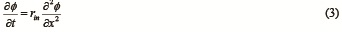Let us redefine the concentration, φ, to be relative to the initial phage concentration, φ0, at time t = 0 such that φr = φ / φ0: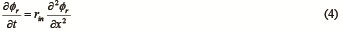Solving for φr, we derive: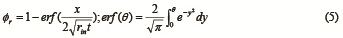The distance of phage diffusion within one time step (i.e. from t = 0 to t = 1) can be estimated by letting φr = 0 and solving for x, which is dependent on the constant, θ, and diffusion coefficient, rin. Note that the integral cannot be solved analytically in closed form. Numerical methods such as the adaptive Simpson quadrature are required to estimate it.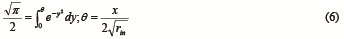Since we are treating this as a one-dimensional problem, the distance of phage diffusion can be roughly estimated by the diffusion length for a time period, dtt: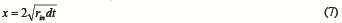For simplicity, we will implement this equation in our simulations.

This distance reflects the depth of the phage-infested layer and is related to the proportion of the total biofilm population susceptible to infection. Details on the incorporation of this equation into the model is discussed in the next section.

Assuming that the volume of the bulk liquid is very large, the concentration of the phage particles in the bulk liquid is negligible and therefore the gradient of phage concentration across the bulk liquid and the phage-infested layer does not affect the diffusion rate into the biofilm. This assumption allows us to estimate the portion of phage particles, δ, in the phage-infested layer that will diffuse out of the biofilm structure: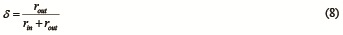This variable influences the decrease term in Equation 2.

### Population Dynamics

The phage population introduced to the boundary layer will infect the biofilm starting at the exposed biofilm surface. A portion of the total biofilm population, ε, is susceptible to infection due to limited phage access to the biofilm biofilm (note that infected bacteria can be re-infected). Because we assume that the composition of biofilm is homogeneous, the portion of susceptible biofilm is related to the depth of phage invasion, z, and the depth of the phage-infested layer, x: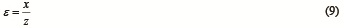The uninfected bacteria and the infected undergo growth at rates, ρu and ρi, respectively. The growth of both subpopulations will contribute to attaining the maximum thickness of the total biofilm. Thus, the dynamics of the two biofilm subpopulations can be described by the following differential equations, where κP cannot exceed εB, the maximum number of biofilm bacteria that can be infected or re-infected: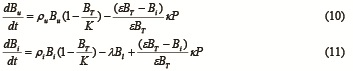The sum of the rate terms of the subpopulations yields the differential equation describing the dynamics of the total bacterial population. Note that the logistic growth component is separated for the subpopulations (Equations 10 and 11). Here, the term for overall biofilm population decline is primarily dependent on the population of bacteria infected with lytic phage, Bl<.

The following differential equations describe the dynamics of the two infected subpopulations, where p is the portion of latent bacteria, π the rate of transition from latent to lytic phase, and λ the rate of host lysis: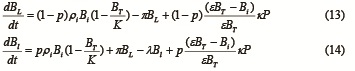Using a simplified geometry of the biofilm structure, we are easily able to describe the dynamic relationship between the depth of the biofilm structure and the total biofilm population: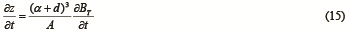### DspB Activity

The production of DspB enzymes is coupled with phage production since they are both activated via the same signal pathway. The concentration of DspB enzymes in the EPS is subject to diffusion forces similar to those acting on the phage particles. The dynamics of extracellular DspB concentration is therefore, where S is the amount of DspB released per host lysis: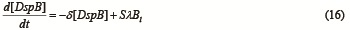The lysis release of DspB enzymes promotes phage invasion by facilitating access into the biofilm structure due to their biofilm EPS-degrading activity. Therefore, DspB increases the diffusion rate of the phage particles into biofilm. The phage particle diffusion rate is related to the concentration of DspB enzymes in the phage-infested layer, where Y is a linear proportionality constant and the rate is constrained by the minimum and maximum values, rmin and rmax, respectively: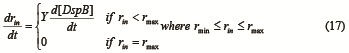### AIP Activity

Here, the biofilm is assumed to maintain its extracellular concentration of AIP at a maximum, [AIP]max. AIP is being secreted by the biofilm bacteria at rate which is a function of the total biofilm population and concentration of AIP and degrades at rate γ. The concentration of AIP is assumed to change more or less logistically: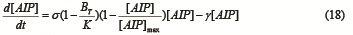The activation of phage production and dspB transcription is dependent on the extracellular concentration of AIP. We engineered the system so that it responds to a certain threshold level of AIP, [AIP]thres, below which phage production and dspB transcription ceases (i.e. R = 0 and S = 0). By modifying Equations 2 and 16, we derive differential equations describing phage population and DspB concentration dynamics conditionally dependent on the concentration of AIP (described by term Z – Equation 21), respectively: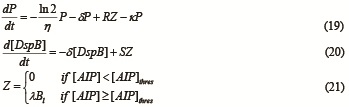### Output

The progress and outcome of phage invasion can be monitored by tracking the populations of the biofilm bacteria and phage. The output of the model can be summarized using the variables BT, Bi, and P:

1) percent of biofilm population remaining since phage introduction (Bp), where B0 is defined as the initial biofilm population or the carrying capacity: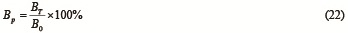2) percent of the biofilm population uninfected by the phage (Binf):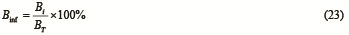3) P-factor (Pf), or the log of the ratio between the phage population and the initial phage population, P0: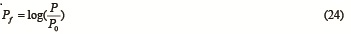### PhilmIt-v1: Model Implementation

A Java implementation of our model (PhilmIt-v1.0) is available for download here. The program has only been tested on Windows 7 (64-bit) and Ubuntu 10.10 (64-bit). The program was written to reflect the modularity of our system. Extensions to our model can be done by altering the equations and/or introducing new terms/equations. Modifications to the program can be easily made by changing the code (please note that if you alter the code, we do not guarantee correct results). Our program can be used as a tool for formulating informed hypotheses for future experiments involving genetically engineered biofilm-phage systems.

### References

1. van Loosdrecht MCM, Heijnen JJ, Eberl H, Kreft J, and Picioreanu C (2002) Mathematical modelling of biofilm structures. Antonie van Leeuwenhoek 81:245-256.

2. Lu and Collins (2007) Dispersing biofilms with engineered enzymatic bacteriophage. Proc Natl Acad Sci 104(27):11197-202.

3. Jepson CD and March JB (2004) Bacteriophage lambda is a highly stable DNA vaccine delivery vehicle. Vaccine 22:2413-19.

4. Otto SP and Day T (2007) A Biologist's Guide to Mathematical Modeling in Ecology and Evolution., Princeton University Press.

5. Smith WF (2004) Foundations of Materials Science and Engineering 3rd ed., McGraw-Hill